# SAT / ACT Prep Online Guides and TipsIn our SAT guide to lines and angles, we dealt with parallel lines, perpendiculars, and the many different ways to find angle measures with two or more lines. Now, we’ll look at the other aspect of lines, namely their slopes and equations.

This will be your complete guide to lines and slopes—what slopes mean, how to find them, and how to solve the many types of slope and line equation questions you’ll see on the SAT.

## What Are Lines and Slopes?

Before we start, you may want to take a moment to familiarize yourself with our guide to SAT coordinate points in order to refresh yourself on the basics of coordinate geometry.

Basically, coordinate geometry takes place in the space where the $x$-axis and the $y$-axis meet. Any place on this space is given a coordinate point—written as $(x, y)$—that indicates where the point is along each axis.

A line (or line segment) is a completely straight marker with no curvature. It is made up of (and connects) a series of points together.

A slope is the measure of the slant (steepness) of a line. A slope is found by finding the change in distance along the y axis over the change in distance along the $x$ axis.

You are probably most familiar with this concept by finding the “rise over run” to find the slope of a line.

$${\change \in y}/{\change \in x}$$Here is a typical line, presented on the coordinate grid. To find our slope, first start by marking the points where the line hits the grid at perfect integer coordinates. This will make life simpler when we go to find the slope.Wherever the grid meets at a corner, we will have coordinates that are integers. We can see here that our line hits the coordinates: $(-3, 4)$, $(0, 2)$, and $(3, 0)$.

Now let us find the rise and run of the line.Our "rise" will be -2, as we must move down 2 units to reach the next coordinate point in our line.

Our run will be +3, as we must move 3 units to the right to reach the next coordinate point in our line.

So our final slope will be:

$\rise/\run$

$-{2/3}$

### Properties of Slopes

A slope can either be positive or negative.

A positive slope rises from left to right.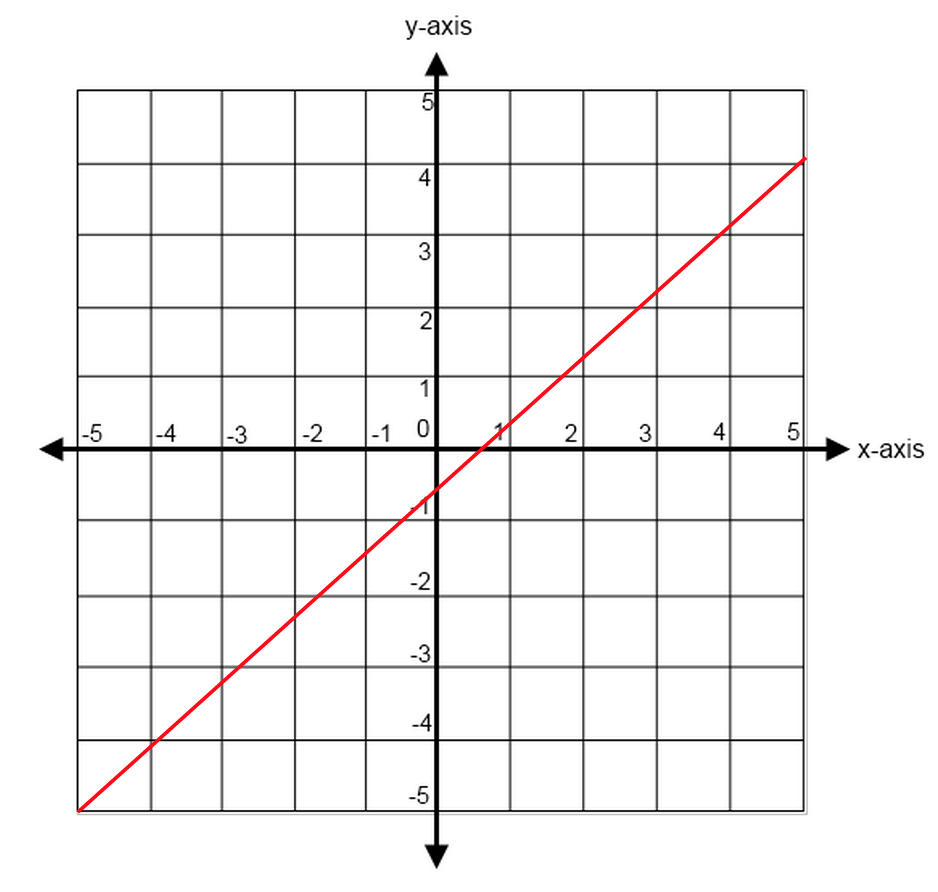A negative slope falls from left to right.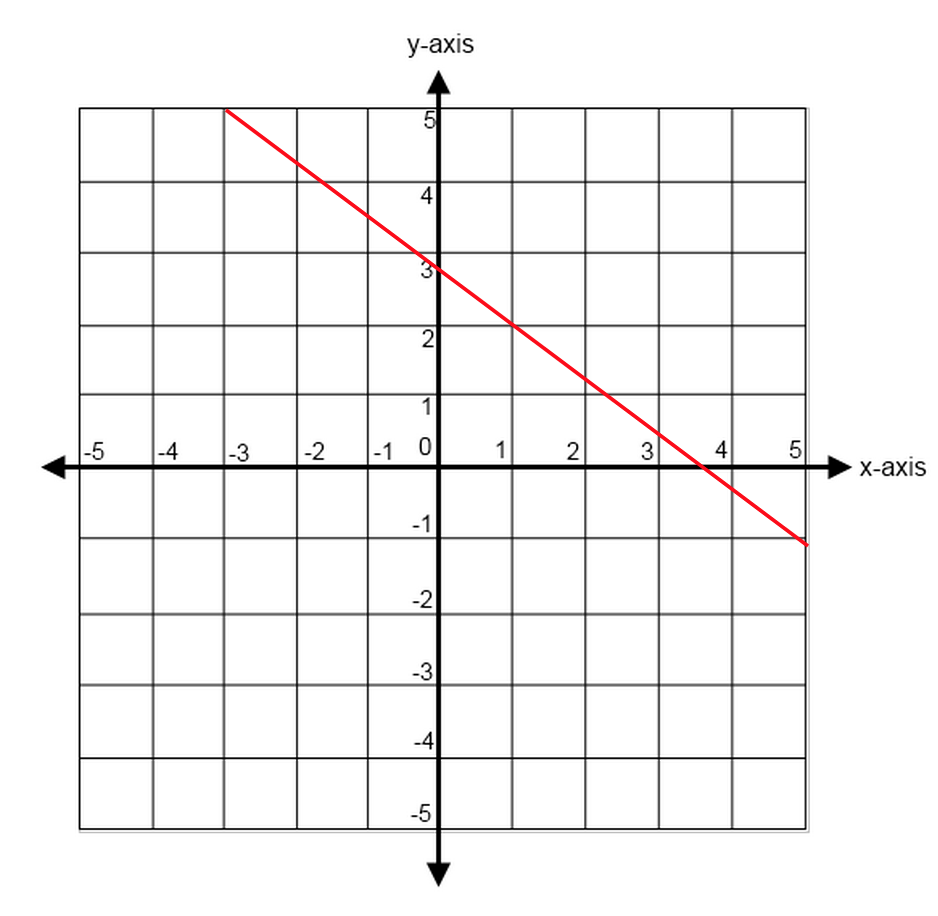A straight horizontal line has a slope of zero. It will be defined by one axis only.$y=2$

A straight vertical line has an undefined slope (because run will always be 0, and you can't divide by 0). It will be defined by one axis only.$x=2.5$

The steeper the line, the larger the slope.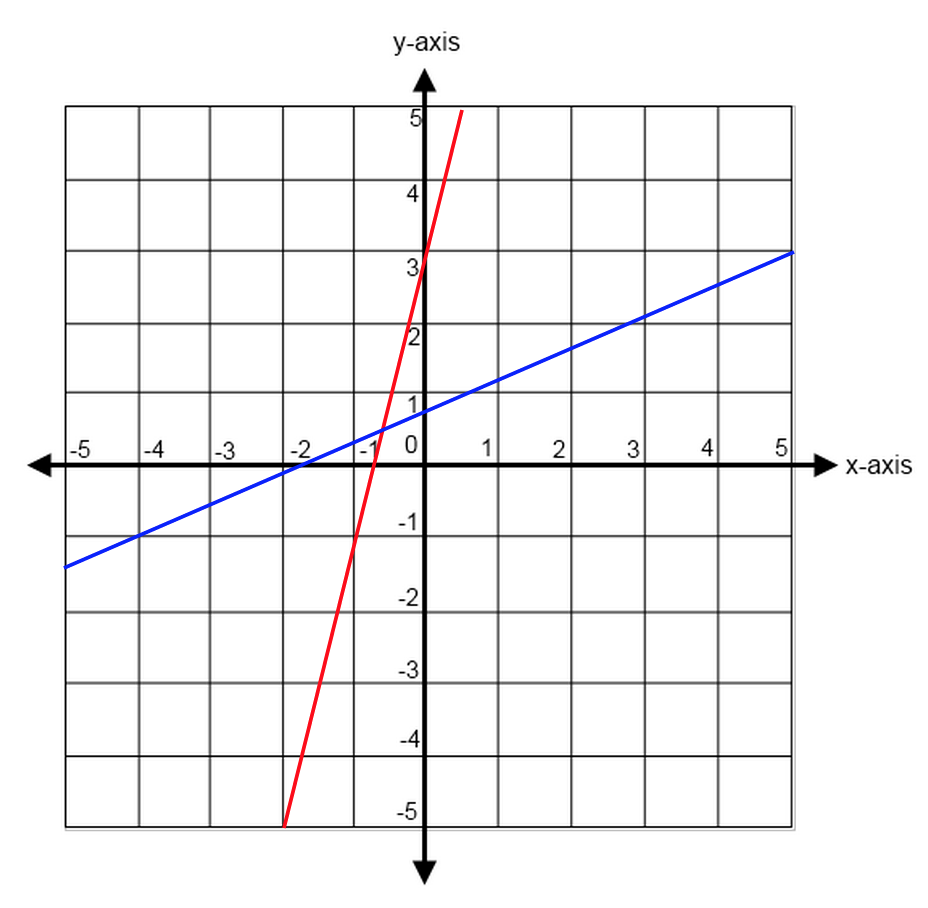The red line is steepest, with a slope of $4/1$, or 4. The blue line is not as steep, with a slope of  $4/9$

##San Francisco knows a little bit about steep slopes.

## Line and Slope Formulas

Finding the Slope

$${y_2 - y_1}/{x_2 - x_1}$$

In order to find the slope of a line that connects two points, you must find the change in the y-values over the change in the x-values.

(Note: It does not matter which points you assign as $(x_1,y_1)$ and $(x_2,y_2)$, so long as you are consistent.)

Given the coordinates $(2, 2)$ and $(-1, 0)$, find the slope of the line.

Now, we can solve this question in one of two ways—by drawing a graph and counting, or by using our formula.Since we already saw earlier how it's possible to count out our slope on a graph, let us use our formula to see how it's done.

Of the two coordinate points, we must assign one set to be $x_1$ and $y_1$ and the other to be $x_2$ and $y_2$. Let us pick (2, 2) to be our $x_1$ and $y_1$ and (-1, 0) to be our $x_2$ and $y_2$.

${y_2 - y_1}/{x_2 - x_1}$

$(0 - 2)/(-1 - 2)$

${-2}/{-3}$

$2/3$

But what would have happened had we assigned $(-1, 0)$ to be our $x_1$ and $y_1$ and $(2, 2)$ to be our $x_2$ and $y_2$? We would have gotten the same results either way!

${y_2 - y_1}/{x_2 - x_1}$

$(2 - 0)/(2 - -1)$

$2/3$

No matter which coordinates we assign to be the first or second values for x and y, we will end up with the same slope so long as we are consistent.

Equation of a Line

$$y=mx+b$$

This is called the “equation of a line” or a line in "slope-intercept form" and it shows exactly how a line is positioned along the $x$- and $y$-axes as well as how steep it is. This is the most important formula you’ll need when it comes to lines and slopes, so let’s break it into pieces.

• $y$ is your $y$-coordinate value for any particular value of $x$.
• $x$ is your $x$-coordinate value for any particular value of $y$.
• $m$ is the measure of your slope.
• $b$ is the $y$-intercept value of your line. This means that it is the value where the line hits the $y$-axis (remember, a straight line will only hit each axis a maximum of one time).

Find the equation of the line from the graph.We're using the same graph from above, and we can see that the line intersects with the $y$-axis at around $y=0.5.$*

We also determined earlier that the slope is $2/3$.

So when we put those two pieces of information together, the equation of our line would be:

$y = {2/3}x + 0.5$

*If the question wants you to be more specific than "about a half" about what fraction of a number the line hits $x=0$ or $y=0$ at, it will have a more detailed drawing. In this question, while the actual intercept for a line with coordinates $(2,2)$ and $(0,-1)$ would actually be $2/3$ or $0.66$, not $0.5$, the graph is not at a scale where you could reasonably visually estimate that.

Remember to always re-write any line equations you are given into the proper form! Often, the test will try to trip you up by giving you an equation not written in proper form and asking for the slope of the line. This is to get people to make a mistake if they are going too quickly through the test.

$$tx+12y=-3$$

The equation above is the equation of a line in the $xy$-plane, and $t$ is a constant. If the slope of the line is -10, what is the value of $t$?

First, let us put this equation into the proper equation of a line.

$tx+12y = −3$

$12y = −tx−3$

$y = - {tx}/{12} -3/12$

Now, we only care about finding the slope, so let’s find the value of t (our slope) by using our given.

$-{t/12} = -10$

$-t = -120$

$t = 120$

Our final answer is $t = 120$.

Always remember to set up your equation as your first step and you’ll be able to solve most any slope problem quickly and easily.

### Perpendicular Lines

When two lines meet at right angles, the lines are called “perpendicular.” Perpendicular lines will always have slopes that are negative reciprocals of one another. This means that you must reverse both the sign of the slope as well as the fraction.

For example, if a two lines are perpendicular to one another and one has a slope of $3/4$ the other line will have a slope of $-{4/3}$.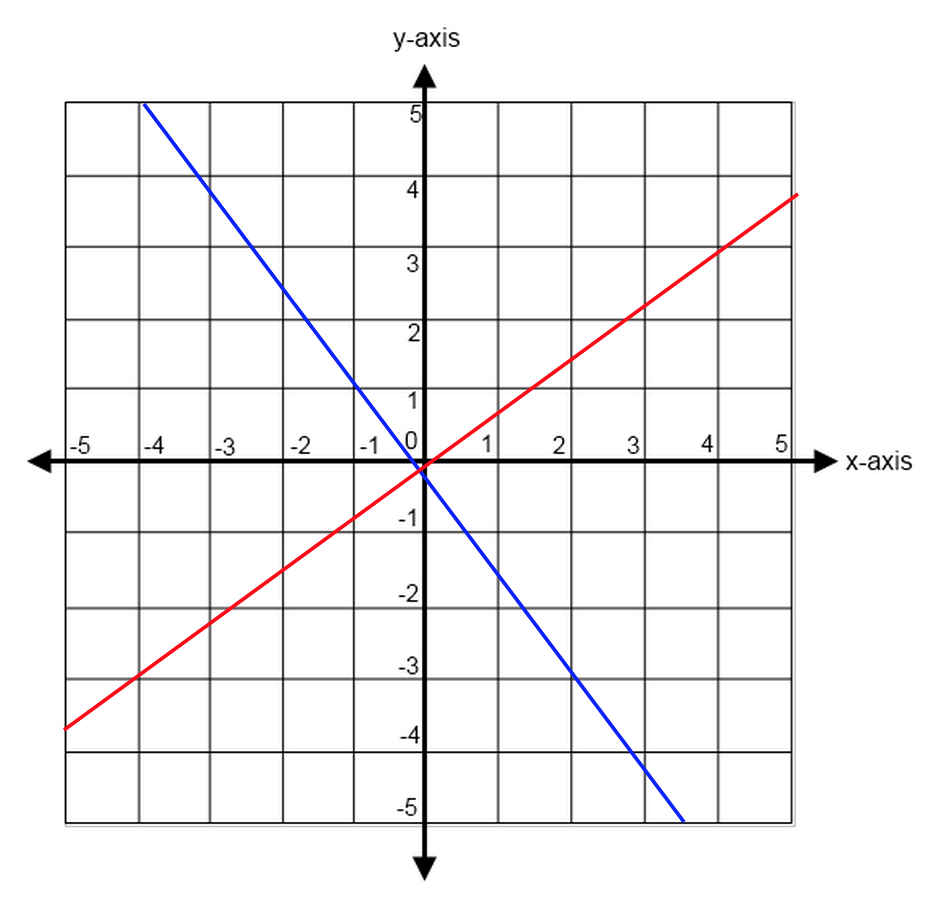Perpendicular lines with slopes of  $3/4$ and $-{4/3}$

And if a line has a slope of −5, the line that meets it perpendicularly will have a slope of $1/5$.

### Parallel Lines

When two lines will never meet, no matter how infinitely long they extend, the lines are said to be parallel. This means that they are continuously equidistant.

If two lines are parallel, they will also have the same slope. You can see why this makes sense, since the rise over run will always have to be the same in order to make sure that the lines will never touch.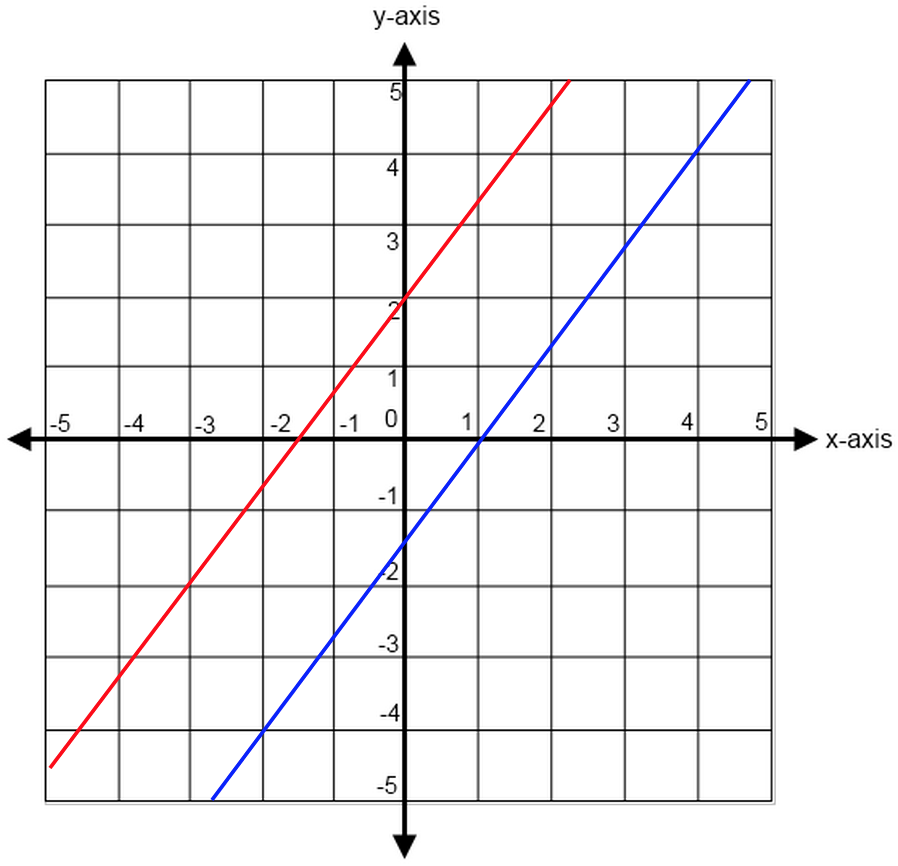Parallel lines with slopes of $4/3$When lines turn devious.

## Typical Line and Slope Questions

Most line and slope questions on the SAT are quite basic at their core. You’ll generally see two questions on slopes per test and almost all of them will simply ask you to find the slope of a line or the equation of a line.

The test may attempt to complicate the question by using other shapes or figures, but the questions always boil down to these simple concepts.

Just remember to re-write any given equations into the proper slope-intercept form and keep in mind your rules for finding slopes (and your rules for parallel or perpendicular lines). With this knowledge in hand, you’ll be able to solve these types of problems quickly and easily.From the graph, we can see that the y-intercept of the line is (0,1).

The line also passes through the point (1,2).

This is enough information for us to figure out the slope of the line, which we know is ${\change \in y}/{\change \in x}$.

${2-1}/{1-0}={1}/{1}=1$

Now we know that the slope of the line is 1.

In slope-intercept form, the equation for line $l$ is $y=x+1$ or choice D.We know that slope is the ${\change \in y}/{\change \in x}$.

The equation $y=kx +4$ is already in slope-intercept form, so we know the slope of the line is $k$.

We also know that the line contains the point $(c,d)$ which means we can substitute those variables for $(x,y)$ in the equation.

This gives us $d=kc+4$

Solving for this equation for the slope, $k$, gives us $k= {d-4}/c$

Our final answer is A, ${d-4}/c$

## How to Solve a Line and Slope Problem

As you go through your line and slope problems, keep in mind these tips:

#1: Always rearrange your equation into $y=mx+b$

The test makers will often present you with an equation of a line that is not in proper form, for example: $4y+3x=12$.

If you are going too quickly through the test or if you forget to rearrange the given equation into proper slope-intercept form, you will misidentify the slope of the line. So always remember to rearrange your equation into proper form as your first step.

$4y + 3x = 12$ => $y = −{3/4}x + 3$

#2: Always remember your $\rise/\run$

It can be easy to make a mistake try to find the change in $x$ before finding the change in y, as our brains are used to doing things "in order." Keep careful track of your variables in order to reduce careless mistakes of this nature.

Remember the mantra of "rise over run" and this will help you always know to find your change in y over your change in $x$.

Because the slope is always "rise over run," you can always find the slope with a graph—whether from a given graph or from your own. It's never a bad idea to take a second and make your own graph if you are not provided with one. This will help you better visualize the problem and avoid errors.

If you forget your formulas (or simply don't want to use them), simply count how the line rises (or falls) and trace its "run," and you will always find your slope.Oh, for the days when all we needed to know about lines was how to color in (or out of) them....

Now that we’ve walked through the typical slope questions you’ll see on the test (and a few basic tips you’ll need to solve them), let’s put your knowledge to the test.

1.2.3.4.1. We know that slope is the ${\change \in y}/{\change \in x}$.

We are also told that our slope is -2, which means it must be $-{2/1}$.

This means that, for every time our y value decreases by 2, our x value increases by 1. And every time our y value increases by 2, our x value will decrease by 1.

If we use our rectangle, we also have one point of reference on the line. We can see that the rectangle has a length of 3 (because it spans horizontally from $x=0$ to $x=3$) and a length of 4 (because it spans vertically from $y=0$ to $y=4$).

This means that the rectangle hits the line at the top right corner at coordinates (3, 4).

Now we can simply count where the line will hit the y-axis.

Because the slope increases horizontally (along the x-axis) one unit at a time, we can see that there will be $3/1 = 3$ even points along the line needed to find the y-intercept. Basically, this means that we are taking the slope, $-{2/1}$, and multiplying it by 3 to get $-{6/3}$.

In other words, we are adding 6 to the change in y and subtracting 3 from the change in x because we are taking the slope backwards.

So now we can find our new point by saying that we increased our y-value from 4 to $4+6=10$, and we have decreased our x-value from 3 to $3−3=0$, which would give us the new slope of:

$(4 + 6)/(3 - 3) = 10/0$

So our new coordinate point is (0, 10), which means that our y-intercept is 10.

Our final answer is D, 10.

2. We know that we can find the slope of the line using ${y_2 - y_1}/{x_2 - x_1}$, so let's plug in our coordinates of (0,r) and (s,0) for these values.

${y_2 - y_1}/{x_2 - x_1}$

$(0 - r)/(s - 0)$

$r/s$

Our s will remain unchanged, but our r value will become negative, as it is being subtracted from zero.

3. If you count to the point at which the line crosses the y-intercept, you can see it hits at $y=3$

In the equation $y=mx+b$, the b is the y-intercept. That means our b will be 3.

We can therefore cross off answer choices A and D, leaving us down to B, C, and E.

We can also see that our line falls from left to right, so our slope will be negative. This means we can eliminate answer choice E, leaving us between choices B and C.

Now let’s take the two points at which the line hits the axes. We already saw that the line hits the y-intercept at $y=3$ and we can also see that the line hits the x-axis at $x=2$. This means our line hits coordinates (0, 3) and (2, 0).

This means that the change in our y value is -3 and the change in our x value is +2. (Why? Because we decreased our y value by 3 and we increased our x value by 2.)

So our slope must be $-{3/2}$, which means that our final equation will be:

$y = -{3/2}x + 3$

4. We're told that the equation of the line is $y=2x-5$.

This means that the slope of the line is 2 and the $y$-intercept is at (0,-5).

A slope of 2 means that, for each increase in $x$ of 1, $y$ increases by 2.

Looking at each of the graphs, choices C and D are the only lines with a slope of 2. (Don't be fooled by choice B which has a slope of -2).

Next we can look at $y$-intercepts.

The line in choice C has a $y$-intercept at ($0,5)$, which doesn't match the equation of the line we were given.

However, choice D's line has a $y$-intercept at $(0,-5)$, which is exactly what we're looking for.

Choice D is the only line with both the correct slope and y-intercept.Hurray, hurray! You've found your slopes, you know your lines! Congrats, congrats.

## The Take-Aways

Once you have familiarized yourself with the basics of coordinate geometry, slopes should not be too far off field. Though the SAT will try to complicate problems as much as they are reasonably able, questions on lines and slopes are almost always easier than they appear.

Keep your important formulas close to your heart and be vigilant with your negative signs, and you’ll do just fine when it comes to slopes and intercepts.

## What’s Next?

Phew! You've learned all there is to learn about your slopes angles. Luckily for you (for a certain definition of "luck"), there is so much more to learn! Before you continue on, make sure you have a firm grasp of all the topics covered on SAT math so that you can see what to prioritize.

If you're looking for a particular math topic, scope out our SAT math archive for individual topic guides like this one. We've got guides to solid geometry, probability, ratios, and more!

If you don't know where to begin, make sure you take a practice test and see how your score ranks. This will give you a good sense of where your strengths and weaknesses are and how you should focus your study time.

Looking to get a perfect score? Check out our guide to getting an 800, written by a perfect scorer!

Want to improve your SAT score by 160 points?

Check out our best-in-class online SAT prep program. We guarantee your money back if you don't improve your SAT score by 160 points or more.

Our program is entirely online, and it customizes what you study to your strengths and weaknesses. If you liked this Math strategy guide, you'll love our program. Along with more detailed lessons, you'll get thousands of practice problems organized by individual skills so you learn most effectively. We'll also give you a step-by-step program to follow so you'll never be confused about what to study next.

Check out our 5-day free trial:Courtney Montgomery

Courtney scored in the 99th percentile on the SAT in high school and went on to graduate from Stanford University with a degree in Cultural and Social Anthropology. She is passionate about bringing education and the tools to succeed to students from all backgrounds and walks of life, as she believes open education is one of the great societal equalizers. She has years of tutoring experience and writes creative works in her free time.

Student and Parent Forum

Our new student and parent forum, at ExpertHub.PrepScholar.com, allow you to interact with your peers and the PrepScholar staff. See how other students and parents are navigating high school, college, and the college admissions process. Ask questions; get answers.